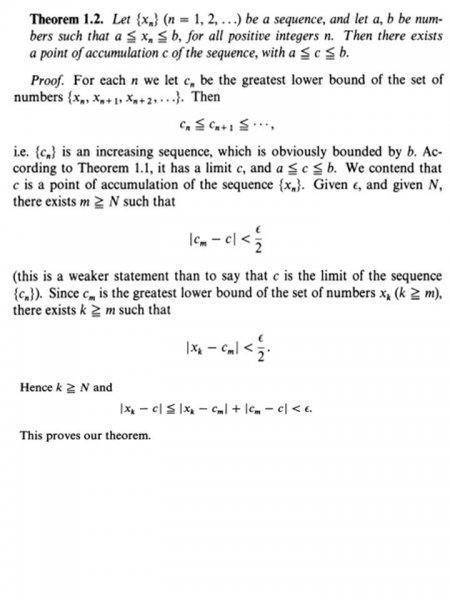# Question about Bolzano-Weierstrass Theorem Proof

• shinobi20
In summary, Serge Lang's proof of the Bolzano-Weierstrass Theorem is odd and difficult to understand. He uses different sources to try to understand the theorem, but eventually fails. The theorem states that every bounded and monotonic sequence is convergent, and Lang's proof relies on this fact. However, his proof does not rely on any other information, and is instead based on the assumption that the Xn's will oscillate randomly. If this assumption is not true, then Cn+1 will not be less than Cn.

#### shinobi20This is the proof of Serge Lang in Undergraduate Analysis. I can't quite understand what he meant in his proof. I read different sources about the theorem but Lang's proof is quite odd. Any help?
BTW. theorem 1.1 just states that Every bounded and monotonic sequence is convergent.

What don't you understand about the proof?

I don't understand the proof of the Bolzano-Weierstrass Theorem according to Serge Lang...

Yes but what about it don't you understand?

How can Cn be increasing? How can we be sure that the Xn will less than Xn+1?

shinobi20 said:
How can Cn be increasing? How can we be sure that the Xn will less than Xn+1?
##C_{n}## is the greatest lower bound of the sequence of ##x_{n}##'s except for the first ##n-1## of them. If you remove some more of the ##x_{n} ##'s then the greatest lower bound can not be less than ##C_{n}##.

Yes but how can we be sure that Cn+1 will not be less than Cn if ever those Xn's oscillate in a very random manner? For example, if Xn+1 is less than Xn, then Cn+1 is the GLB of the set Xn+1's, and Cn is the GLB of the Xn's but this implies Cn+1 is less than Cn.

shinobi20 said:
Yes but how can we be sure that Cn+1 will not be less than Cn if ever those Xn's oscillate in a very random manner? For example, if Xn+1 is less than Xn, then Cn+1 is the GLB of the set Xn+1's, and Cn is the GLB of the Xn's but this implies Cn+1 is less than Cn.
Because they are greatest lower bounds. It doesn't matter if the X's oscillate. ##C_{n}## is lower than all of them except the first ##n-1##. ##C_{n+1}## is lower than all of them except one less so that one removed might be very low.

Oh! Now I got it, because I was thinking that Cn's can overlap or surpass the Xn+1's and vice versa... Thanks!